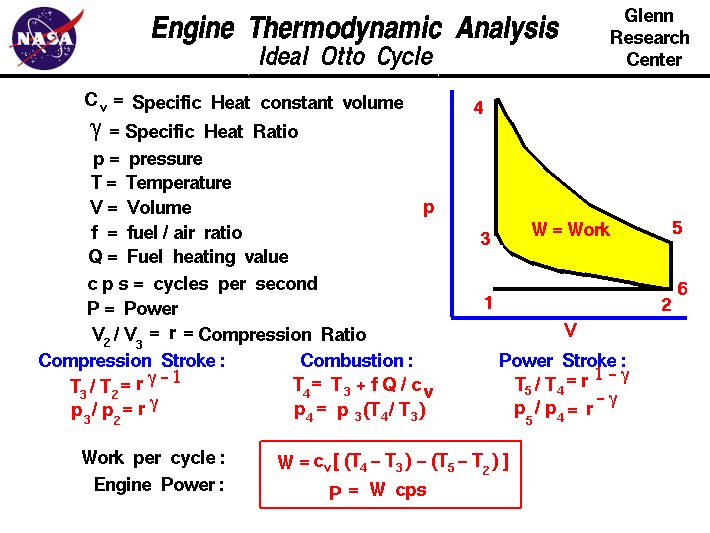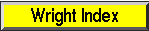+ Text Only Site
+ Non-Flash Version
+ Contact GlennThermodynamics is a branch of physics which deals with the energy and work of a system. It was born in the 19th century as scientists were first discovering how to build and operate steam engines. Thermodynamics deals only with the large scale response of a system which we can observe and measure in experiments. As aerodynamicists, we are most interested in the thermodynamics of propulsion systems and high speed flows. On this page we consider the thermodynamics of a four-stroke internal combustion engine. Today, most general aviation or private airplanes are powered by internal combustion (IC) engines, much like the engine in your family automobile. There are two main parts to engine operation: the mechanical operation of the engine parts, and the thermodynamics through which the engine produces work and power. On this page we discuss the basic thermodynamic equations that allow you to design and predict engine performance. In an internal combustion engine, fuel and air are ignited inside a cylinder. The hot exhaust pushes a piston which is connected to a crankshaft to produce power. The burning of fuel is not a continuous process, but occurs very quickly at regular time intervals. Between ignitions, the engine parts move in a repeated sequence called a cycle. The engine is called a four stroke engine because there are four movements, or strokes, of the piston during one cycle. On the figure we show a plot of pressure versus gas volume throughout one cycle. We have broken the cycle into six numbered stages based on the mechanical operation of the engine. For the ideal four stroke engine, the intake stroke (1-2) and exhaust stroke (6-1) are done at constant pressure and do not contribute to the generation of power by the engine. During the compression stroke (2-3), work is done on the gas by the piston. If we assume that no heat enters the gas during the compression, we know the relations between the change in volume and the change in pressure and temperature from our solutions of the entropy equation for a gas. We call the ratio of the volume at the beginning of compression to the volume at the end of compression the compression ratio, r. Then p3 / p2 = r ^ gamma T3 / T2 = r ^ (gamma - 1) where p is the pressure, T is the temperature, and gamma is the ratio of specific heats. During the combustion process (3-4), the volume is held constant and heat is released. The change in temperature is given by T4 = T3 + f * Q /cv where Q is the heat released per pound of fuel which depends on the fuel,f is the fuel/air ratio for combustion which depends on several factors associated with the design and temperature in the combustion chamber, and cv is the specific heat at constant volume. From the equation of state, we know that: p4 = p3 * (T4 /T3) During the power stroke (4-5), work is done by the gas on the piston. The expansion ratio is the reciprocal of the compression ratio and we can use the same relations used during the compression stroke: p5 / p4 = r ^ (-gamma) T5 / T4 = r ^ (1 - gamma) Between stage 5 and stage 6, residual heat is transferred to the surroundings so that the temperature and pressure return to the initial conditions of stage 1 (or 2). During the cycle, work is done on the gas by the piston between stages 2 and 3. Work is done by the gas on the piston between stages 4 and 5. The difference between the work done by the gas and the work done on the gas is shown in yellow and is the work produced by the cycle. We can calculate the work by determining the area enclosed by the cycle on the p-V diagram. But since the processes 2-3 and 4-5 are curves, this is a difficult calculation. We can also evaluate the work W by the difference of the heat into the gas minus the heat rejected by the gas. Knowing the temperatures, this is an easier calculation. W = cv * [(T4 - T3) - (T5 - T2)] The work times the rate of the cycle (cycles per second cps) is equal to the power P produced by the engine. P = W * cps On this page we have shown an ideal Otto cycle in which there is no heat entering (or leaving) the gas during the compression and power strokes, no friction losses, and instantaneous burning occurring at constant volume. In reality, the ideal cycle does not occur and there are many losses associated with each process. These losses are normally accounted for by efficiency factors which multiply and modify the ideal result. For a real cycle, the shape of the p-V diagram is similar to the ideal, but the area (work) is always less than the ideal value. Activities: Guided Tours Navigation ..Beginner's Guide Home Page+ Inspector General Hotline + Equal Employment Opportunity Data Posted Pursuant to the No Fear Act + Budgets, Strategic Plans and Accountability Reports + Freedom of Information Act + The President's Management Agenda + NASA Privacy Statement, Disclaimer, and Accessibility CertificationEditor: Nancy Hall NASA Official: Nancy Hall Last Updated: May 13 2021 + Contact Glenn